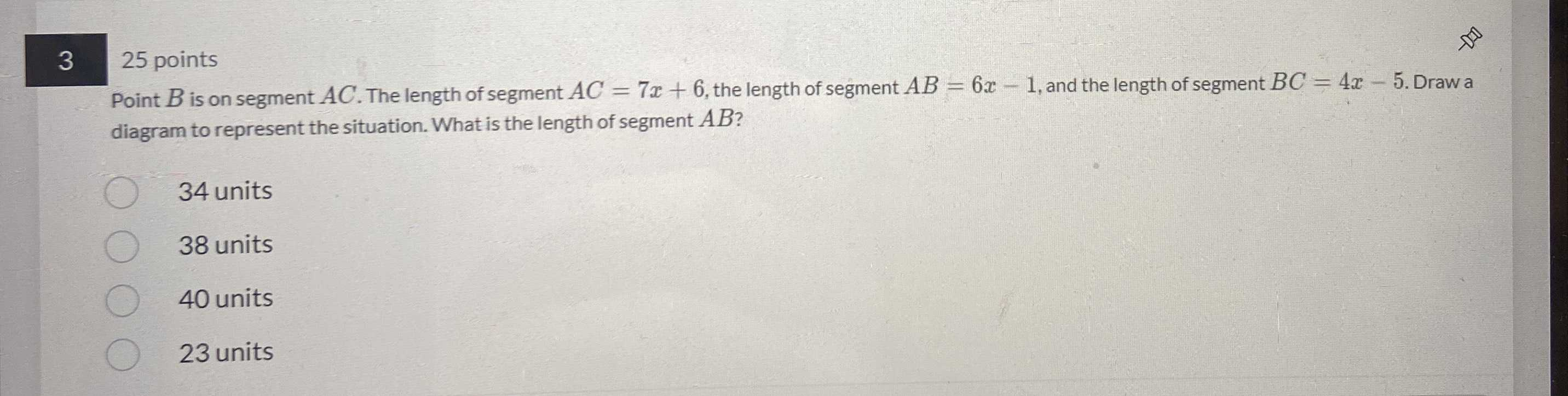### Still have math questions?

Algebra
Question$$325$$ points Point $$B$$ is on segment $$A C$$ . The length of segment $$A C = 7 x + 6$$ , the length of segment $$A B = 6 x - 1$$ , and the length of segment $$B C = 4 x - 5$$ . Draw a diagram to represent the situation. What is the length of segment $$A B$$ ?

$$34$$ units

$$38$$ units

$$40$$ units

$$23$$ units